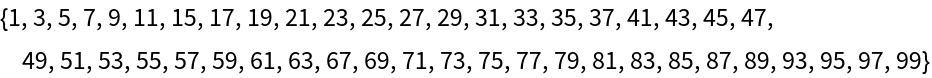Function Repository Resource:

AffineDecipher

Decipher a string using the affine cipher

Contributed by: Sander Huisman
 ResourceFunction["AffineDecipher"][string,{a,b}] deciphers string using the affine cipher with parameters a and b. ResourceFunction["AffineDecipher"][{a,b}] represents an operator form of ResourceFunction["AffineDecipher"] that can be applied to an expression.

Details and Options

For the affine cipher the letters az are numbered 0–25. Each character in string is converted to a number (x) using the aforementioned numbering. Each number is then transformed by computing (ax+b) mod 26. The new numbers are then reinterpreted as letters. Here is an example enciphering the letters AZ for a=5 and b=8. Deciphering is done by reading the diagram from bottom to top.The case of the letters is retained by ResourceFunction["AffineDecipher"].

Examples

Basic Examples

Convert a simple text:

 In:=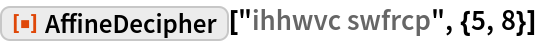Out=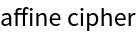Scope

AffineDecipher automatically maps over a list of strings:

 In:=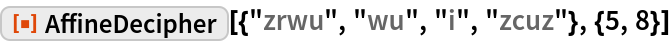Out=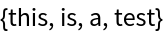Create an operator:

 In:=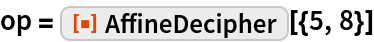Out=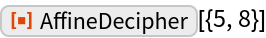Apply the operator to a string:

 In:=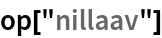Out=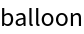Properties and Relations

The Caesar decipher can be recreated using the affine decipher by choosing a=1:

 In:=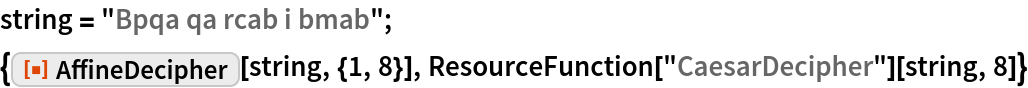Out=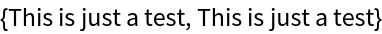AffineDecipher could be recast in terms of ResourceFunction["AffineCipher"]:

 In:=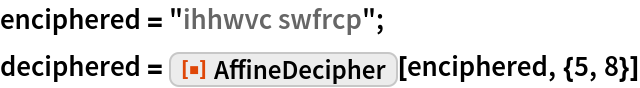Out=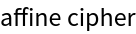Again decipher, this time using AffineCipher with appropriate parameter values for inverting:

 In:=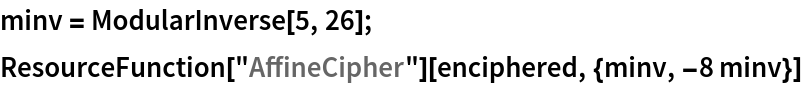Out=Possible Issues

The parameter a must be coprime to 26:

 In:=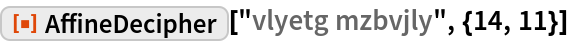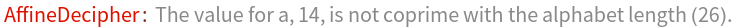Out=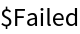Some of the permissible values for a are:

 In:=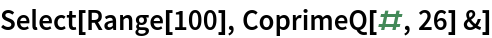Out=